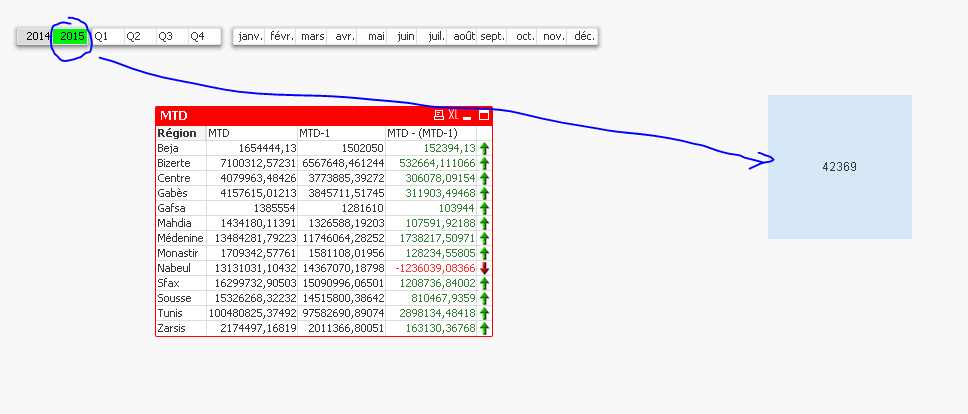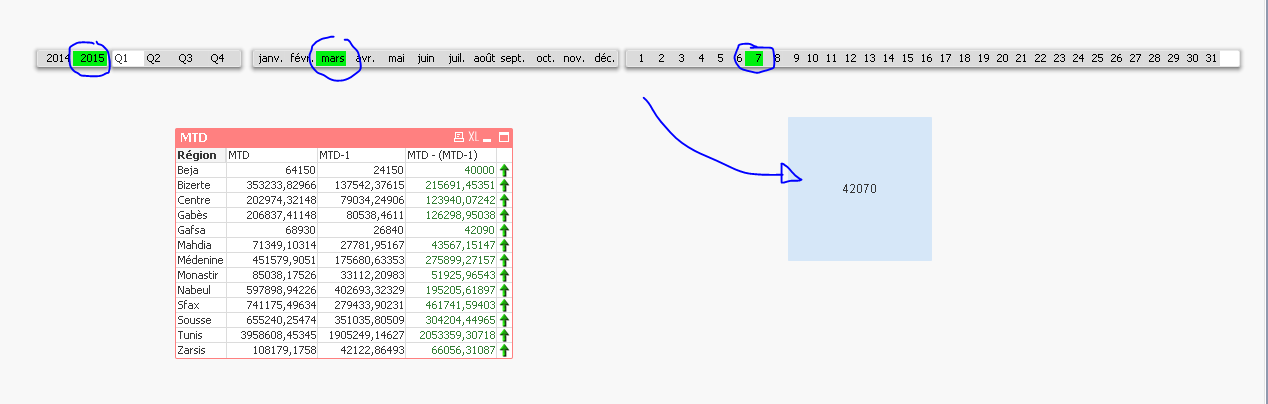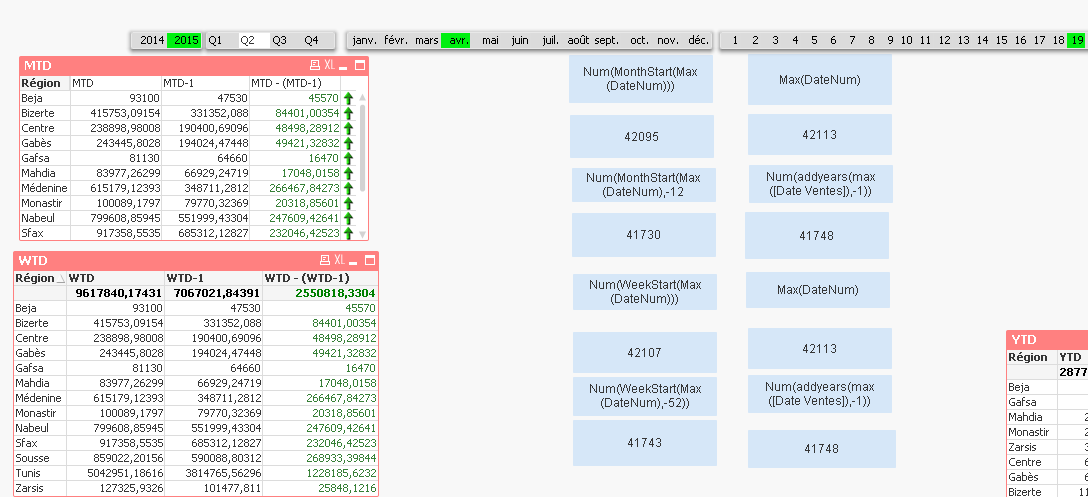# New to QlikView

Discussion board where members can get started with QlikView.

HighlightedPartner

## Problem with week to date expression

Hi all,

I have a trouble with the WeekToDate expression.

In fact, the values returned when I use the WTD are the same returned with the MTD..

As if the WeekStart function acts like the MonthStart function and returns the debut of each month.

Here are the expressions I used for each case:

WTD:

Sum({<Year=, Month=, Quarter=, [Date Ventes]= , DateNum={">=\$(=Num(WeekStart(Max(DateNum))))<=\$(=Max(DateNum))"}>} [Chiffre d'affaires])

WTD-1 :

Sum({<Year=, Month=, Quarter=, [Date Ventes]= , DateNum={">=\$(=Num(WeekStart(Max(DateNum),-52)))<=\$(=Num(addyears(max([Date Ventes]),-1)))"}>} [Chiffre d'affaires]

MTD :

Sum({<Year=, Month=, Quarter=, [Date Ventes]= , DateNum={">=\$(=Num(MonthStart(Max(DateNum))))<=\$(=Max(DateNum))"}>} [Chiffre d'affaires])

MTD-1 :

Sum({<Year=, Month=, Quarter=, [Date Ventes]= , DateNum={">=\$(=Num(MonthStart(Max(DateNum),-12)))<=\$(=Num(addyears(max([Date Ventes]),-1)))"}>} [Chiffre d'affaires])

I have yet another question: what's the purpose of "Year=, Month=, Quarter=, [Date Ventes]=" ? I know that if I delete them and then filter by dte, I won't have the same result. But why?

Thank you !

Tags (2)
10 RepliesMVP

## Re: Problem with week to date expression

Is your Max(DateNum) happens to be Feb 2nd 2016? Because if that is the case then you MTD and WTD will be exactly the same because there is 2 days WTD (1st and 2nd Feb) and 2 days in MTD (1st and 2nd Feb)

To answer your second question, this is how you specify which field selections should not change anything in the chart. The issue is that if you don't add them, Date or Month selection will further filter out from your date range specified in the DateNum field. You don't want that to happen so you restrict any other date field selection and allow it to move just on DateNum

Honored Contributor II

## Re: Problem with week to date expression

Why do you deduct 52? Think you need to have the Weeknumber of the previous year?

The command "Year=, Month=, Quarter=, [Date Ventes]=" means that the function shall disregard all entries made in these fields.

PeterPartner

## Re: Problem with week to date expression

No, the max(dateNum) returns the num of the date I select to filter with. For example,Partner

## Re: Problem with week to date expression

Yes, I think the year contains 52 weeks; so I deduct 52, It would happen to be the same week of the previous year, no?Partner

## Re: Problem with week to date expression

I added the Max to have a result even though I didn't make a selection

Honored Contributor II

## Re: Problem with week to date expression

From the above code it seems that in your expression

.... DateNum={">=\$(=Num(WeekStart(Max(DateNum),-52)))....

DateNum is a Date and the whole expression refers to a date, so deducting 52 ends with a date approx 2 monthes in the past.

For the calculation with weeks it seems to be easier to work with rolling weeks, like

Year(MyDate)*52 + Week(MyDate)     AS WeekNum

Doing so might make your life a bit easier

PeterMVP

## Re: Problem with week to date expression

For the WTD-1, I would probably use AddYears also in the lower limit:

though this selection will show one to two days less dates than WTD, so may want to modify the upper limit.

can't see why WTD and MTD should return same values (unless for specific DateNum selections, as mentioned above.

If you remove the labels of your chart expressions, then hover with the mouse over the labels, the expression will be shown with dollar sign expansions expanded. What do the two expressions show in the field modifier ranges?Partner

## Re: Problem with week to date expression

Here is a screen shot that resumes the confusing part ! The expression i'm using are correct, the result should differ but I keep on having the same result !!I really I'm confused !Partner

## Re: Problem with week to date expression

here is another example :